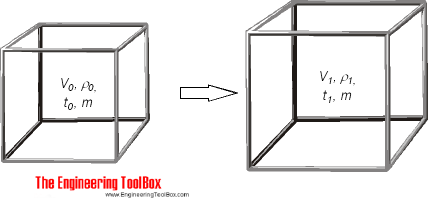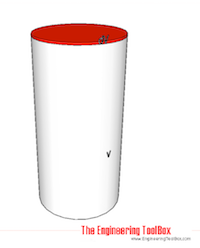Engineering ToolBox - Resources, Tools and Basic Information for Engineering and Design of Technical Applications!

# Volumetric (Cubic) Thermal Expansion

## Volumetric temperature expansion calculator.Specific volume of a unit can be expressed as

v = 1 / ρ = V / m                                                          (1)

where

v = specific volume (m3/kg, ft3/lb)

ρ = density (kg/m3, lb/ft3)

V = volume of unit (m3, ft3)

m = mass of unit (kg, lb)

The change in the units volume when temperature changes can be expressed as

dV = V0 β (t1 - t0)                                                        (2)

where

dV = V1 - V0 = change in volume (m3, ft3)

β = volumetric temperature expansion coefficient (m3/m3 oC, ft3/ft3 oF)

t1 = final temperature (oC, oF)

t0 = initial temperature (oC, oF)

The density of a fluid when temperature changes can be expressed as

ρ1 = m / V0 (1 + β (t1 - t0))

= ρ0 / (1 + β (t1 - t0))                                                (3)

where

ρ1 = final density (kg/m3, lb/ft3)

ρ0 = initial density (kg/m3, lb/ft3)

### Online Thermal Cubic Expansion Calculator - Expansion coefficient and Temperatures

Be aware that the expansion coefficient for some liquids - like water - may vary with temperature. The calculator below is generic and can be used for metric and imperial units as long as the use of units is consistent.

V0 - initial volume (m3, ft3, gallons ..)

β - volumetric temperature expansion coefficient (1/oC, 1/oF)

t0 - initial temperature (oC, oF)

t1 - final temperature (oC, oF)

Note that the volumetric expansion coefficient used in the calculator is constant. If you want to calculate volumetric change for a liquid over a temperature range where the volumetric expansion coefficient for the liquid changes a lot - interpolate the coefficient values, or split the calculation in the different temperature ranges. Example - water is a liquid where the volumetric expansion coefficient changes a lot with temperature. Water has its highest density and smallest volume at 4oC (39.2 oF). The volumetric coefficient for water is negative below 4oC and indicates that the a volume decreases when temperature moves from 0oC (32 oF) to 4oC.

### Online Thermal Cubic Expansion Calculator - Densities

This calculator can be used to calculate expansion volume when initial volume and initial and final densities for the liquid are known

V0 - initial volume (m3, ft3)

ρ0 - initial density (kg/m3, lb/ft3)

ρ1 - final density (kg/m3, lb/ft3)

### Volumetric Temperature Coefficients - β - for some Fluids

• water at 0oC: -0.000050 (1/oC)
• water at 4oC: 0 (1/oC)
• water at 10oC: 0.000088 (1/oC)
• water at 20oC: 0.000207 (1/oC)
• water at 30oC: 0.000303 (1/oC)
• water at 40oC: 0.000385 (1/oC)
• water at 50oC: 0.000457 (1/oC)
• water at 60oC: 0.000522 (1/oC)
• water at 70oC: 0.000582 (1/oC)
• water at 80oC: 0.000640 (1/oC)
• water at 90oC: 0.000695 (1/oC)
• ethyl alcohol: 0.00109 (1/ oC), 0.00061 (1/oF)
• oil: 0.00070 (1/oC), 0.00039 (1/oF)

### Convert between Metric and Imperial Volumentric Temperature Coefficients

• 1 (1/oC) =  0.56 (1/oF)
• 1 (1/oF) =  1.8 (1/oC)

### Example - Cubic Expansion of Oil100 liters - 0.1 m3 - of oil with volumetric expansion coefficient 0.00070 1/oC is heated from 20oC to 40oC. The volumetric expansion can be calculated using equation (2)

dV = (0.1 m3) (0.00070 1/oC) ((40 oC) - (20 oC))

= 0.0014 m3

= 1.4 liter

The final volume is

100 liters + 1.4 liters = 101.4 liters

### Example - Cubic Expansion of Oil

30 US gallons of oil is heated from 70oF to 100oF. The volumetric expansion can be calculated using equation (2)

dV = (30 gallons) (0.00039 1/oF) ((100 oF) - (70 oF))

= 0.351 gallons

The final volume is

30 gallons + 0.351 gallon = 30.351 gallons

## Related Topics

• Heating - Heating systems - capacity and design of boilers, pipelines, heat exchangers, expansion systems and more.
• Water Systems - Hot and cold water service systems - design properties, capacities, sizing and more.
• Thermodynamics - Work, heat and energy systems.
• Densities - Densities of solids, liquids and gases. Definitions and convertion calculators.

## Engineering ToolBox - SketchUp Extension - Online 3D modeling!

Add standard and customized parametric components - like flange beams, lumbers, piping, stairs and more - to your Sketchup model with the Engineering ToolBox - SketchUp Extension - enabled for use with the amazing, fun and free SketchUp Make and SketchUp Pro .Add the Engineering ToolBox extension to your SketchUp from the SketchUp Pro Sketchup Extension Warehouse!

Translate

## Privacy

We don't collect information from our users. Only emails and answers are saved in our archive. Cookies are only used in the browser to improve user experience.

Some of our calculators and applications let you save application data to your local computer. These applications will - due to browser restrictions - send data between your browser and our server. We don't save this data.

## Citation

• Engineering ToolBox, (2009). Volumetric (Cubic) Thermal Expansion. [online] Available at: https://www.engineeringtoolbox.com/volumetric-temperature-expansion-d_315.html [Accessed Day Mo. Year].

Modify access date.

. .

#### Scientific Online Calculator3 10